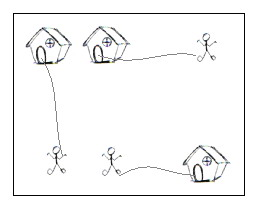# Competitive Programming: POJ 2195 – Going Home

### Description

On a grid map there are n little men and n houses. In each unit time, every little man can move one unit step, either horizontally, or vertically, to an adjacent point. For each little man, you need to pay a \$1 travel fee for every step he moves, until he enters a house. The task is complicated with the restriction that each house can accommodate only one little man.

Your task is to compute the minimum amount of money you need to pay in order to send these n little men into those n different houses. The input is a map of the scenario, a ‘.’ means an empty space, an ‘H’ represents a house on that point, and am ‘m’ indicates there is a little man on that point.You can think of each point on the grid map as a quite large square, so it can hold n little men at the same time; also, it is okay if a little man steps on a grid with a house without entering that house.

### Input

There are one or more test cases in the input. Each case starts with a line giving two integers N and M, where N is the number of rows of the map, and M is the number of columns. The rest of the input will be N lines describing the map. You may assume both N and M are between 2 and 100, inclusive. There will be the same number of ‘H’s and ‘m’s on the map; and there will be at most 100 houses. Input will terminate with 0 0 for N and M.

### Output

For each test case, output one line with the single integer, which is the minimum amount, in dollars, you need to pay.

```2 2
.m
H.
5 5
HH..m
.....
.....
.....
mm..H
7 8
...H....
...H....
...H....
mmmHmmmm
...H....
...H....
...H....
0 0
```

### Sample Output

```2
10
28
```

Solution below . . .

```/*
* The Hungarian algorithm (https://en.wikipedia.org/wiki/Hungarian_algorithm)
* can be used to solve assignment problems. Given an NxN matrix, where the
* element in the i-th row and j-th column represents the cost of assigning
* the i-th house to the j-th man, the algorithm finds an assignment of men
* to houses with minimum cost.
*/

import java.util.ArrayList;
import java.util.Arrays;
import java.util.List;
import java.util.Scanner;

public class Main {

// a[1..n][1..m] >= 0, n <= m
public static int solveAssignmentProblem(int&#91;&#93;&#91;&#93; a) {
int n = a.length - 1;
int m = a&#91;0&#93;.length - 1;

int&#91;&#93; u = new int&#91;n + 1&#93;;
int&#91;&#93; v = new int&#91;m + 1&#93;;
int&#91;&#93; p = new int&#91;m + 1&#93;;
int&#91;&#93; way = new int&#91;m + 1&#93;;

for (int i = 1; i <= n; ++i) {
p&#91;0&#93; = i;
int j0 = 0;
int&#91;&#93; minv = new int&#91;m + 1&#93;;

Arrays.fill(minv, Integer.MAX_VALUE);

boolean&#91;&#93; used = new boolean&#91;m + 1&#93;;

do {
used&#91;j0&#93; = true;

int i0 = p&#91;j0&#93;;
int delta = Integer.MAX_VALUE;
int j1 = 0;

for (int j = 1; j <= m; ++j)
if (!used&#91;j&#93;) {
int cur = a&#91;i0&#93;&#91;j&#93; - u&#91;i0&#93; - v&#91;j&#93;;
if (cur < minv&#91;j&#93;) {
minv&#91;j&#93; = cur;
way&#91;j&#93; = j0;
}
if (minv&#91;j&#93; < delta) {
delta = minv&#91;j&#93;;
j1 = j;
}
}
for (int j = 0; j <= m; ++j)
if (used&#91;j&#93;) {
u&#91;p&#91;j&#93;&#93; += delta;
v&#91;j&#93; -= delta;
} else
minv&#91;j&#93; -= delta;
j0 = j1;
} while (p&#91;j0&#93; != 0);

do {
int j1 = way&#91;j0&#93;;
p&#91;j0&#93; = p&#91;j1&#93;;
j0 = j1;
} while (j0 != 0);
}
return -v&#91;0&#93;;
}

public static void main(String&#91;&#93; args) {

List<Pair<Integer, Integer>> men;
List<Pair<Integer, Integer>> houses;

Pair<Integer, Integer> p;

Scanner sc = new Scanner(System.in);

int N = sc.nextInt();
int M = sc.nextInt();

while (N != 0) {

men = new ArrayList<Pair<Integer, Integer>>();
houses = new ArrayList<Pair<Integer, Integer>>();

for (int i = 0; i < N; i++) {
String s = sc.next();

for (int j = 0; j < M; j++) {
char c = s.charAt(j);

if (c == 'm') {
p = new Pair<Integer, Integer>(i, j);
}

if (c == 'H') {
p = new Pair<Integer, Integer>(i, j);
}
}
}

int count = houses.size();
int[][] a = new int[count + 1][count + 1];

for (int i = 1; i <= count; i++) {
Pair<Integer, Integer> p1 = houses.get(i - 1);

for (int j = 1; j <= count; j++) {
Pair<Integer, Integer> p2 = men.get(j - 1);

a[i][j] = distance(p1, p2);
}
}

System.out.println(solveAssignmentProblem(a));

N = sc.nextInt();
M = sc.nextInt();
}

sc.close();
}

static int distance(Pair<Integer, Integer> p1, Pair<Integer, Integer> p2) {
return Math.abs(p1.e1 - p2.e1) + Math.abs(p1.e2 - p2.e2);
}
}

class Pair<S, T> {
final S e1;
final T e2;

Pair(final S e1, final T e2) {
this.e1 = e1;
this.e2 = e2;
}
}
```